Скачать презентацию Inflation November 8 2010 Inflation can be

f6de5ff292d94e3d8c13112d1bbf1c78.ppt

• Количество слайдов: 38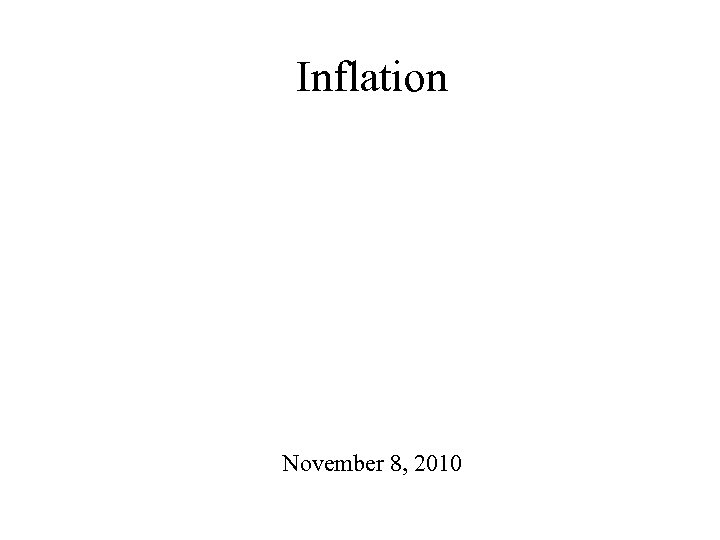Inflation November 8, 2010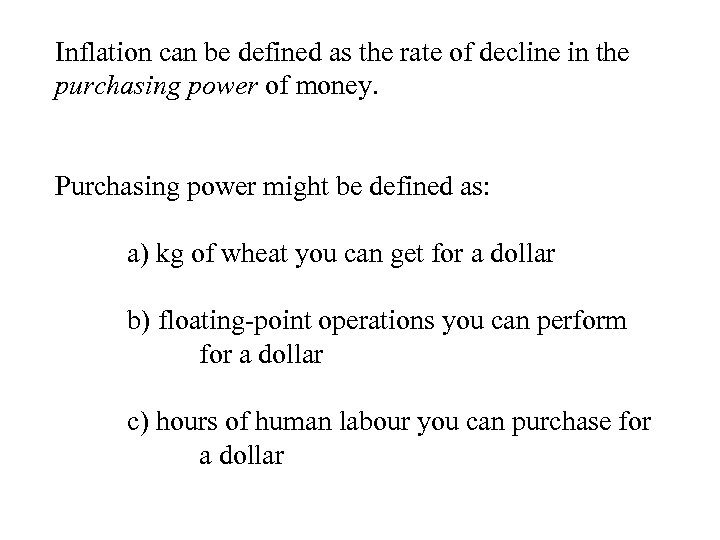Inflation can be defined as the rate of decline in the purchasing power of money. Purchasing power might be defined as: a) kg of wheat you can get for a dollar b) floating-point operations you can perform for a dollar c) hours of human labour you can purchase for a dollar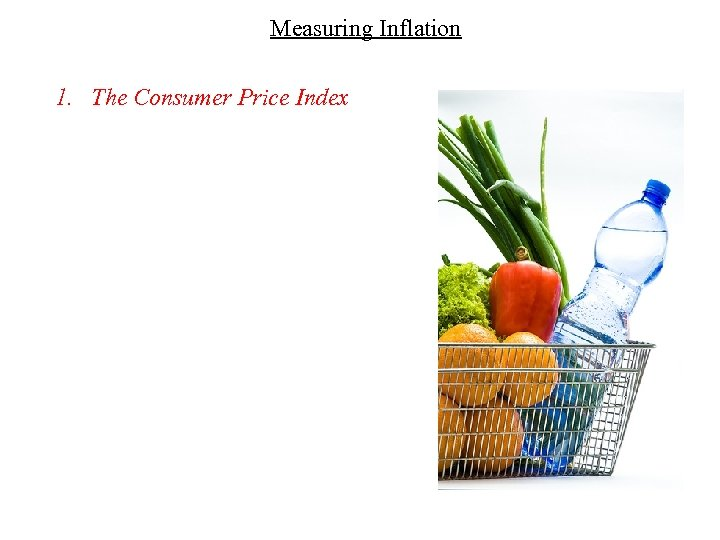Measuring Inflation 1. The Consumer Price Index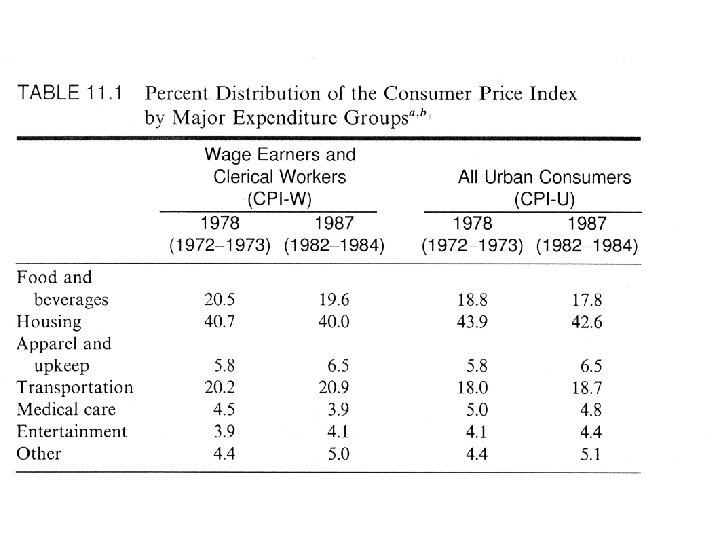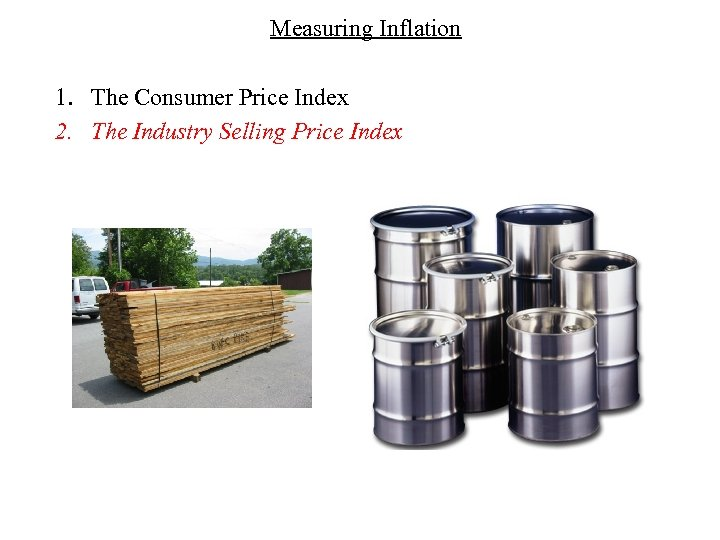Measuring Inflation 1. The Consumer Price Index 2. The Industry Selling Price Index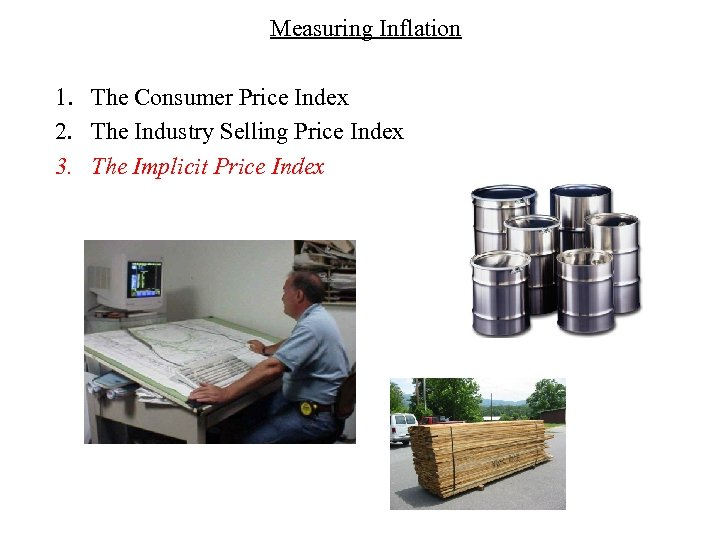Measuring Inflation 1. The Consumer Price Index 2. The Industry Selling Price Index 3. The Implicit Price Index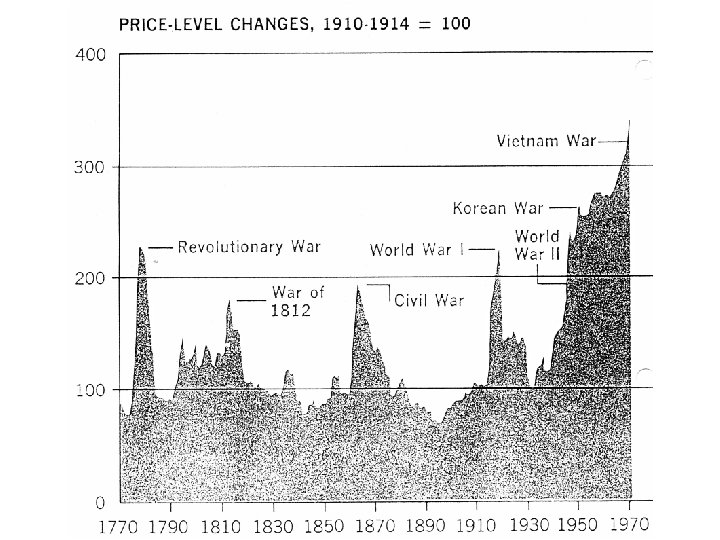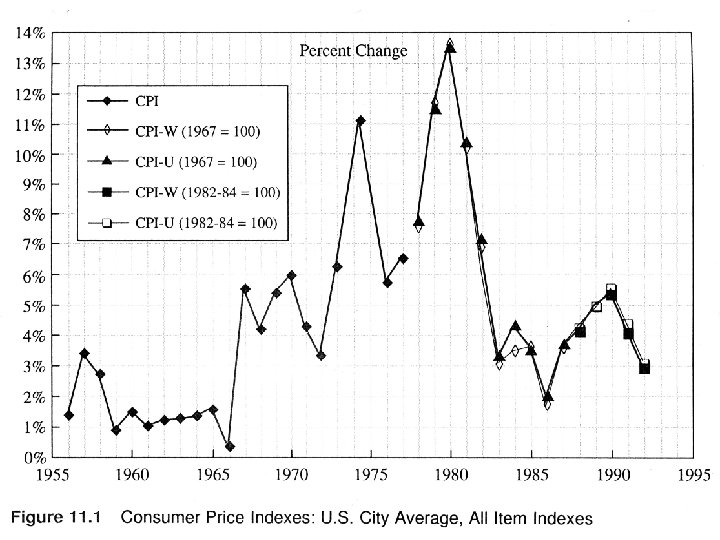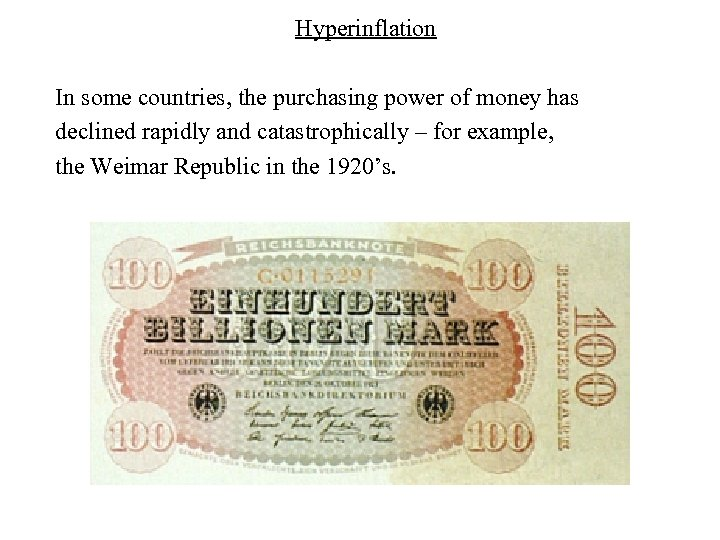Hyperinflation In some countries, the purchasing power of money has declined rapidly and catastrophically – for example, the Weimar Republic in the 1920’s.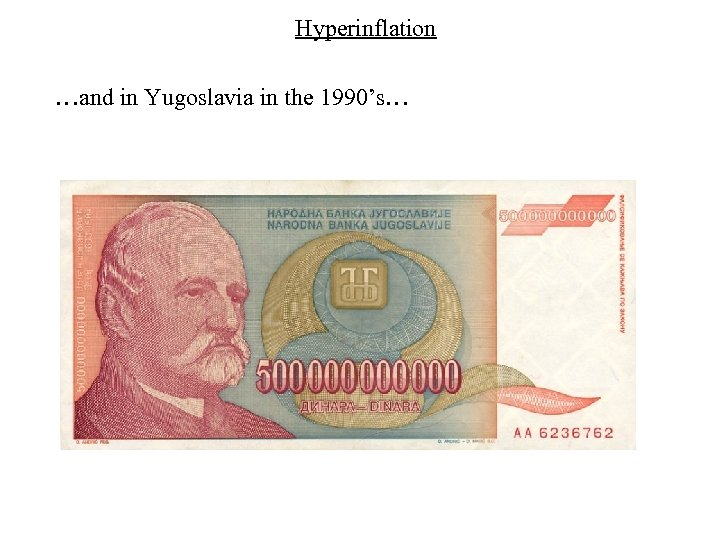Hyperinflation …and in Yugoslavia in the 1990’s…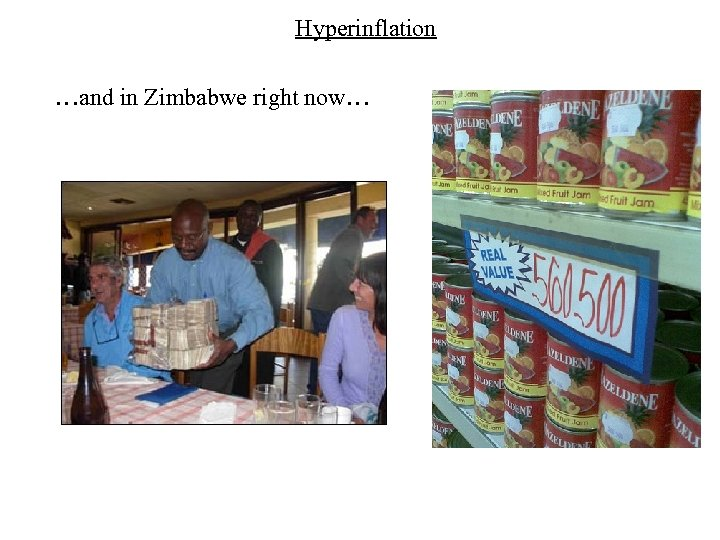Hyperinflation …and in Zimbabwe right now…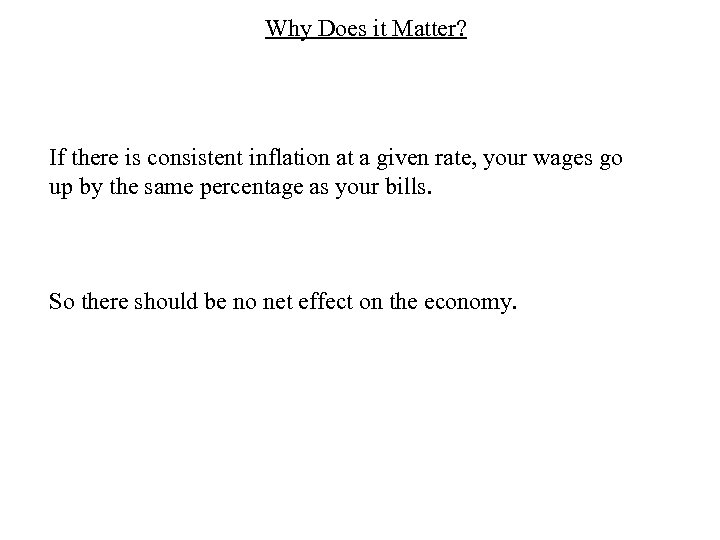Why Does it Matter? If there is consistent inflation at a given rate, your wages go up by the same percentage as your bills. So there should be no net effect on the economy.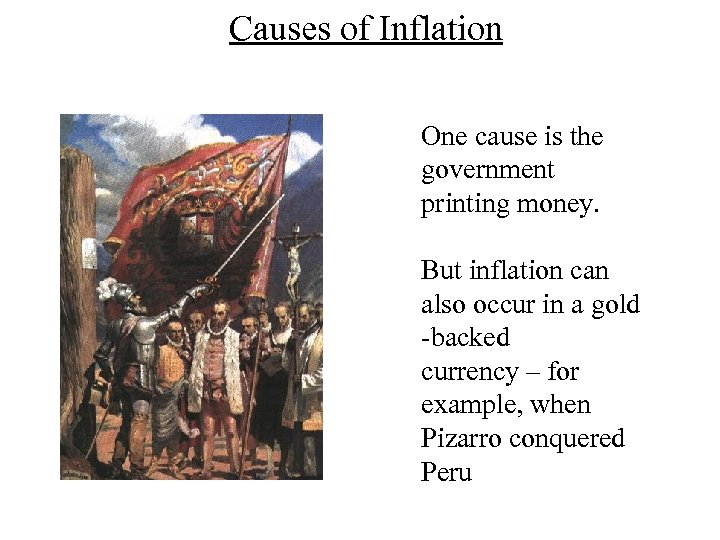Causes of Inflation One cause is the government printing money. But inflation can also occur in a gold -backed currency – for example, when Pizarro conquered Peru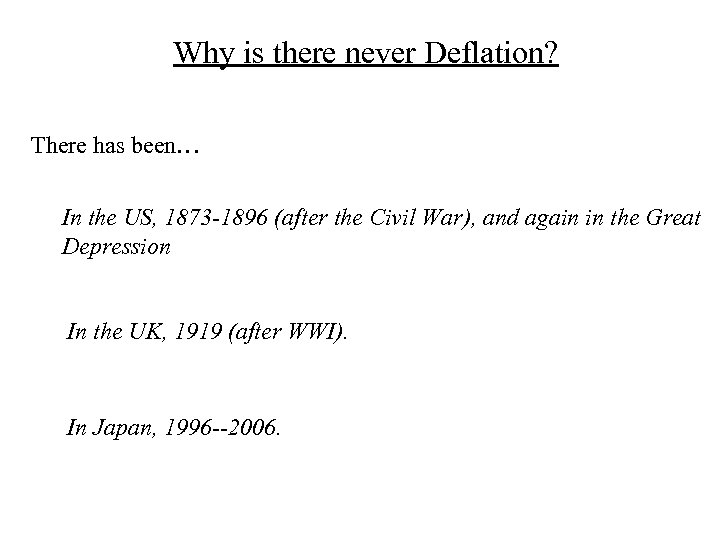Why is there never Deflation? There has been… In the US, 1873 -1896 (after the Civil War), and again in the Great Depression In the UK, 1919 (after WWI). In Japan, 1996 --2006.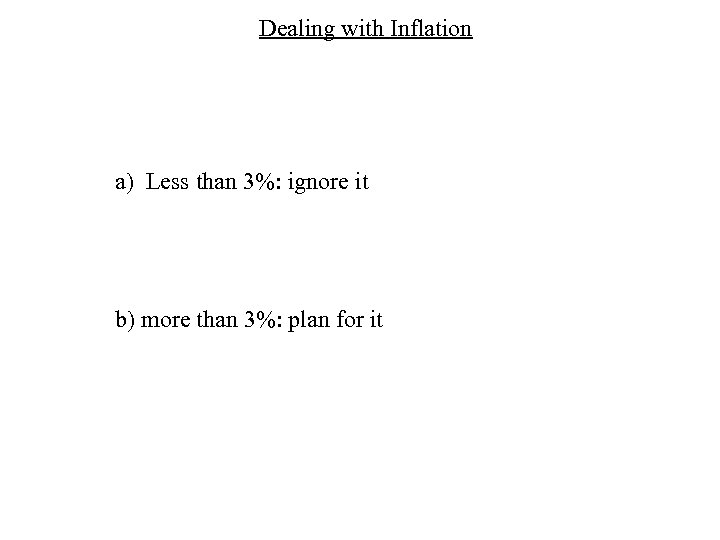Dealing with Inflation a) Less than 3%: ignore it b) more than 3%: plan for it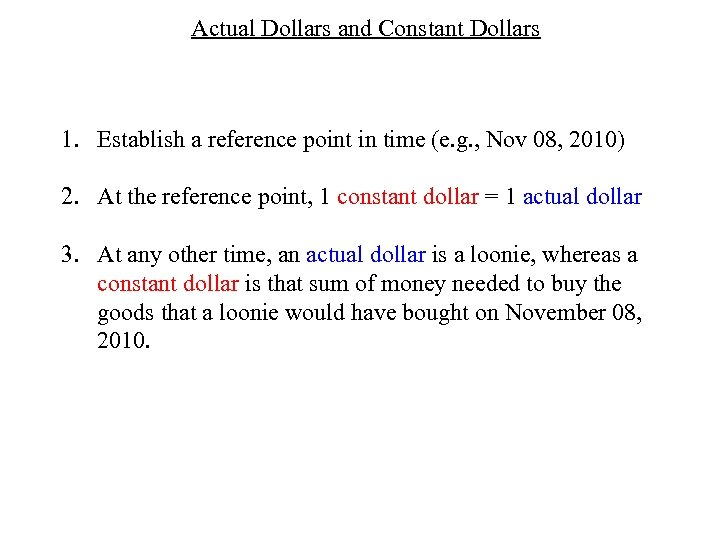Actual Dollars and Constant Dollars 1. Establish a reference point in time (e. g. , Nov 08, 2010) 2. At the reference point, 1 constant dollar = 1 actual dollar 3. At any other time, an actual dollar is a loonie, whereas a constant dollar is that sum of money needed to buy the goods that a loonie would have bought on November 08, 2010.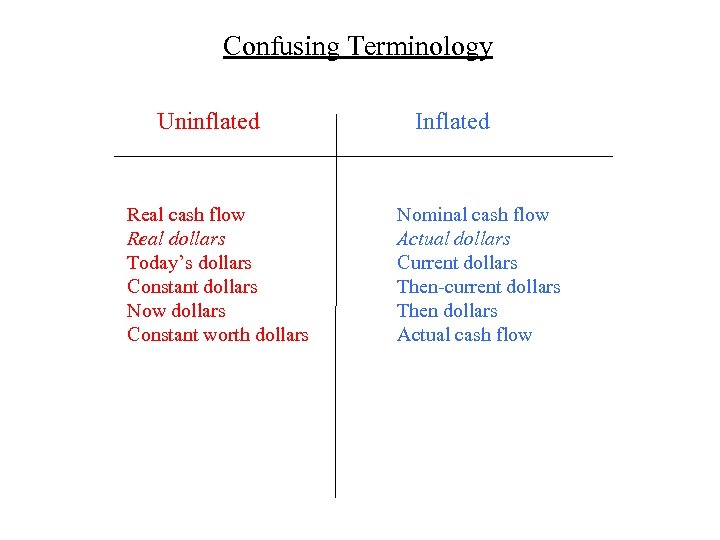Confusing Terminology Uninflated Real cash flow Real dollars Today’s dollars Constant dollars Now dollars Constant worth dollars Inflated Nominal cash flow Actual dollars Current dollars Then-current dollars Then dollars Actual cash flow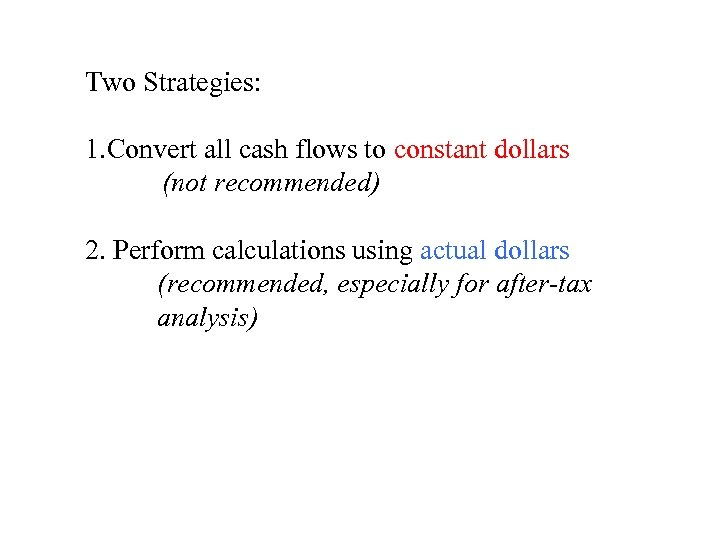Two Strategies: 1. Convert all cash flows to constant dollars (not recommended) 2. Perform calculations using actual dollars (recommended, especially for after-tax analysis)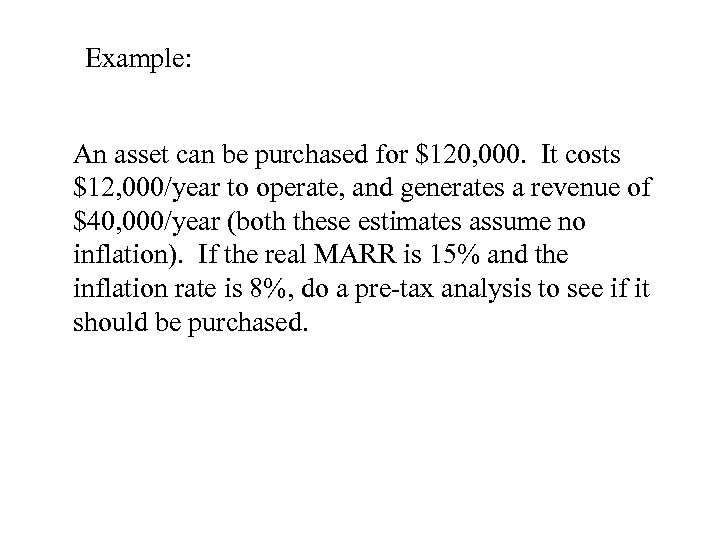Example: An asset can be purchased for \$120, 000. It costs \$12, 000/year to operate, and generates a revenue of \$40, 000/year (both these estimates assume no inflation). If the real MARR is 15% and the inflation rate is 8%, do a pre-tax analysis to see if it should be purchased.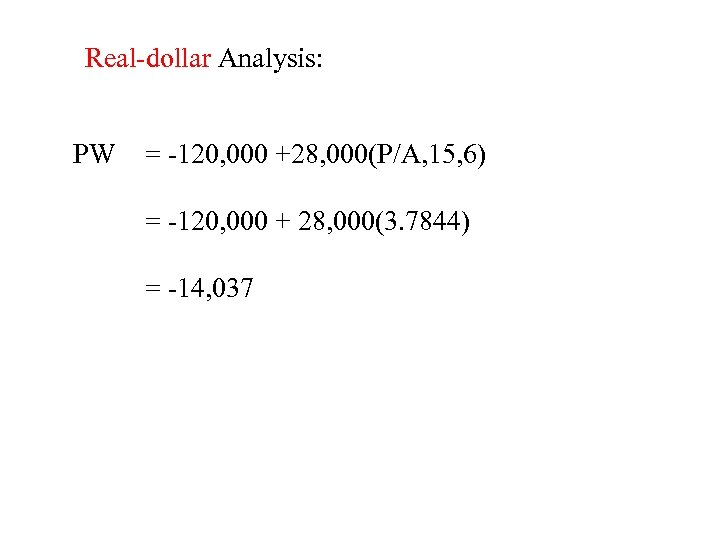Real-dollar Analysis: PW = -120, 000 +28, 000(P/A, 15, 6) = -120, 000 + 28, 000(3. 7844) = -14, 037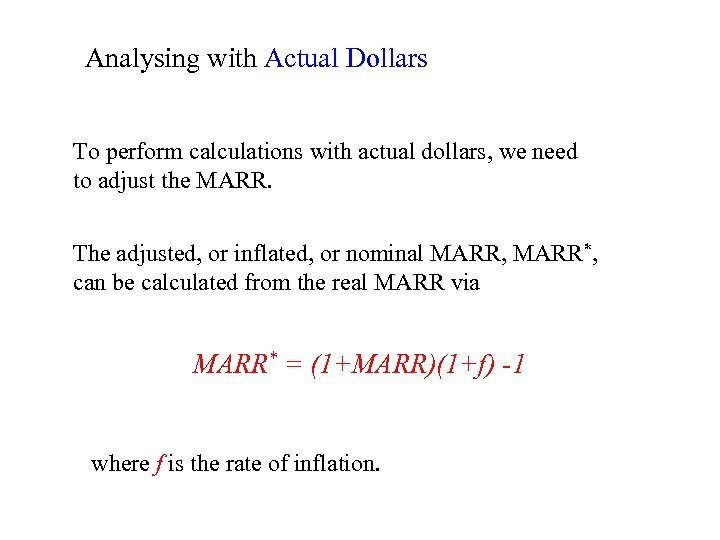Analysing with Actual Dollars To perform calculations with actual dollars, we need to adjust the MARR. The adjusted, or inflated, or nominal MARR, MARR*, can be calculated from the real MARR via MARR* = (1+MARR)(1+f) -1 where f is the rate of inflation.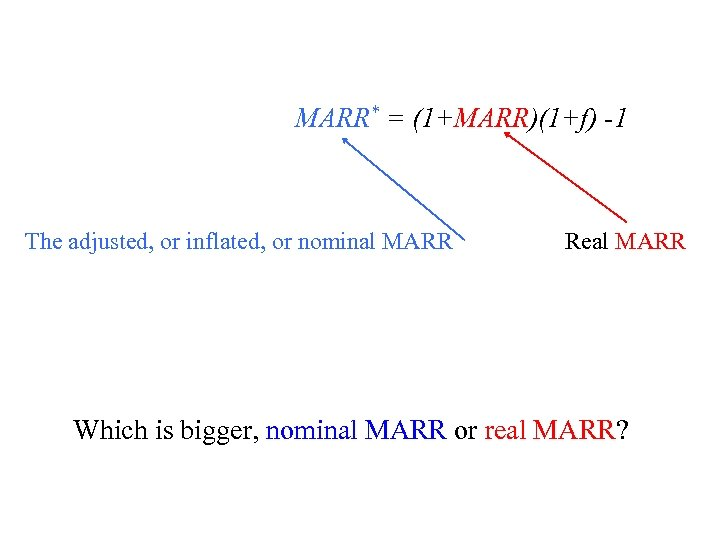MARR* = (1+MARR)(1+f) -1 The adjusted, or inflated, or nominal MARR Real MARR Which is bigger, nominal MARR or real MARR?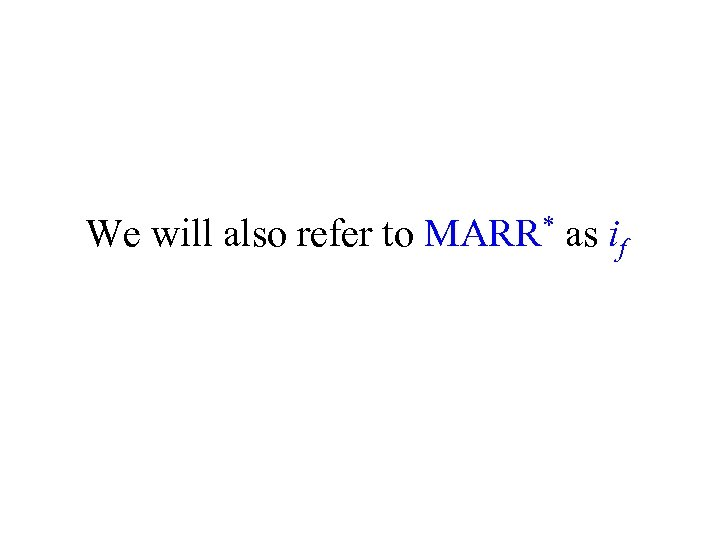We will also refer to MARR* as if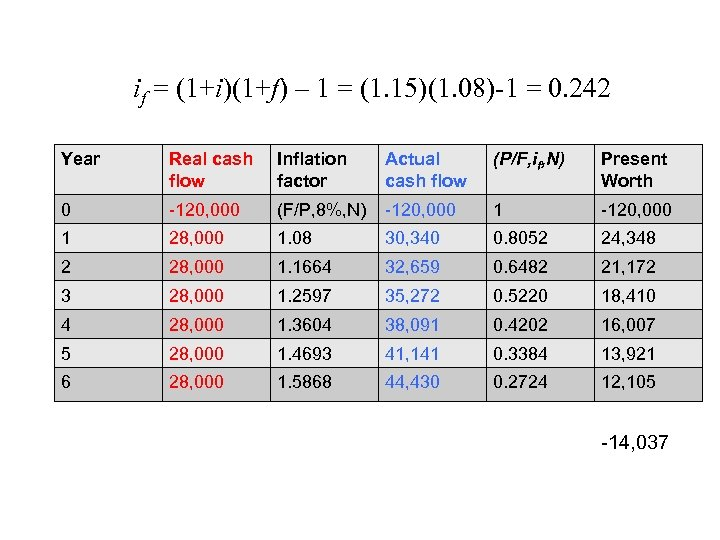if = (1+i)(1+f) – 1 = (1. 15)(1. 08)-1 = 0. 242 Year Real cash flow Inflation factor Actual cash flow (P/F, if, N) Present Worth 0 -120, 000 (F/P, 8%, N) -120, 000 1 28, 000 1. 08 30, 340 0. 8052 24, 348 2 28, 000 1. 1664 32, 659 0. 6482 21, 172 3 28, 000 1. 2597 35, 272 0. 5220 18, 410 4 28, 000 1. 3604 38, 091 0. 4202 16, 007 5 28, 000 1. 4693 41, 141 0. 3384 13, 921 6 28, 000 1. 5868 44, 430 0. 2724 12, 105 -14, 037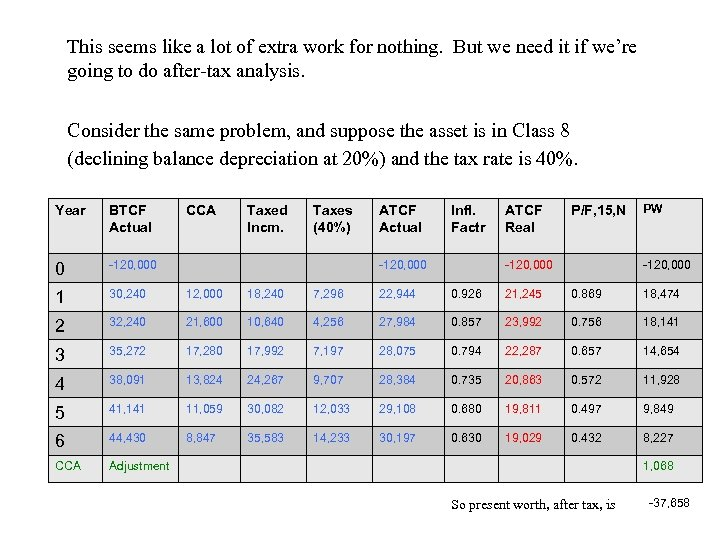This seems like a lot of extra work for nothing. But we need it if we’re going to do after-tax analysis. Consider the same problem, and suppose the asset is in Class 8 (declining balance depreciation at 20%) and the tax rate is 40%. CCA Taxed Incm. Taxes (40%) ATCF Actual Infl. Factr BTCF Actual 0 -120, 000 1 30, 240 12, 000 18, 240 7, 296 22, 944 0. 926 21, 245 0. 869 18, 474 2 32, 240 21, 600 10, 640 4, 256 27, 984 0. 857 23, 992 0. 756 18, 141 3 35, 272 17, 280 17, 992 7, 197 28, 075 0. 794 22, 287 0. 657 14, 654 4 38, 091 13, 824 24, 267 9, 707 28, 384 0. 735 20, 863 0. 572 11, 928 5 41, 141 11, 059 30, 082 12, 033 29, 108 0. 680 19, 811 0. 497 9, 849 6 44, 430 8, 847 35, 583 14, 233 30, 197 0. 630 19, 029 0. 432 8, 227 CCA Adjustment -120, 000 ATCF Real P/F, 15, N PW Year -120, 000 1, 068 So present worth, after tax, is -37, 658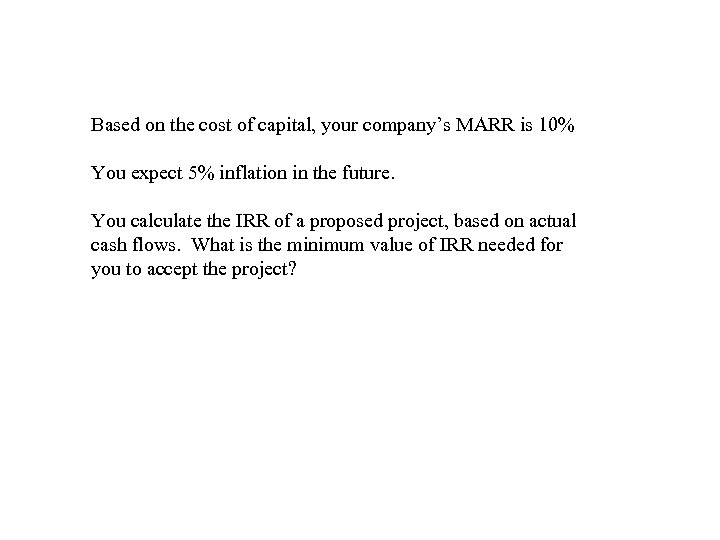Based on the cost of capital, your company’s MARR is 10% You expect 5% inflation in the future. You calculate the IRR of a proposed project, based on actual cash flows. What is the minimum value of IRR needed for you to accept the project?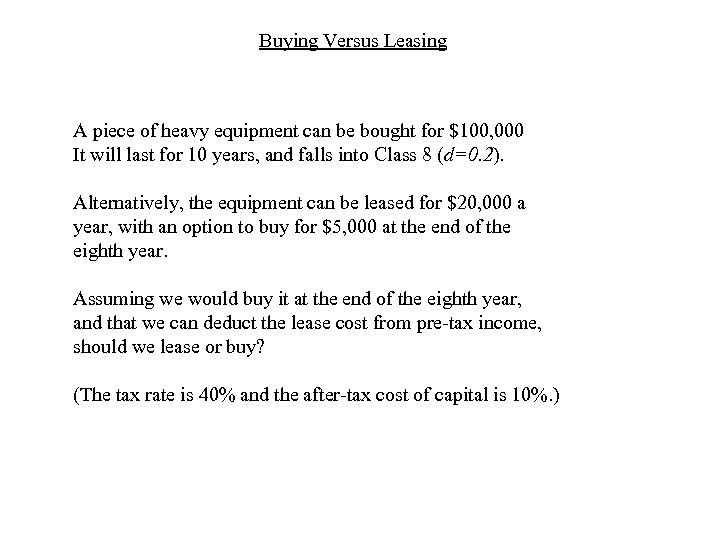Buying Versus Leasing A piece of heavy equipment can be bought for \$100, 000 It will last for 10 years, and falls into Class 8 (d=0. 2). Alternatively, the equipment can be leased for \$20, 000 a year, with an option to buy for \$5, 000 at the end of the eighth year. Assuming we would buy it at the end of the eighth year, and that we can deduct the lease cost from pre-tax income, should we lease or buy? (The tax rate is 40% and the after-tax cost of capital is 10%. )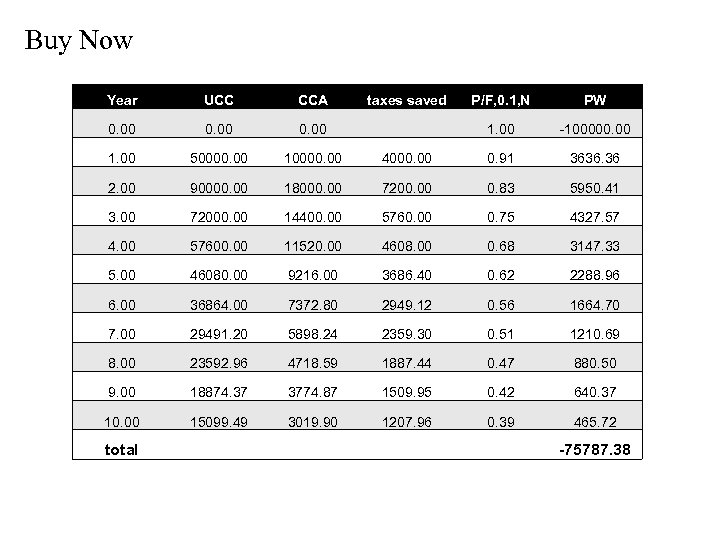Buy Now Year UCC CCA 0. 00 1. 00 50000. 00 10000. 00 2. 00 90000. 00 3. 00 P/F, 0. 1, N PW 1. 00 -100000. 00 4000. 00 0. 91 3636. 36 18000. 00 7200. 00 0. 83 5950. 41 72000. 00 14400. 00 5760. 00 0. 75 4327. 57 4. 00 57600. 00 11520. 00 4608. 00 0. 68 3147. 33 5. 00 46080. 00 9216. 00 3686. 40 0. 62 2288. 96 6. 00 36864. 00 7372. 80 2949. 12 0. 56 1664. 70 7. 00 29491. 20 5898. 24 2359. 30 0. 51 1210. 69 8. 00 23592. 96 4718. 59 1887. 44 0. 47 880. 50 9. 00 18874. 37 3774. 87 1509. 95 0. 42 640. 37 10. 00 15099. 49 3019. 90 1207. 96 0. 39 465. 72 total taxes saved -75787. 38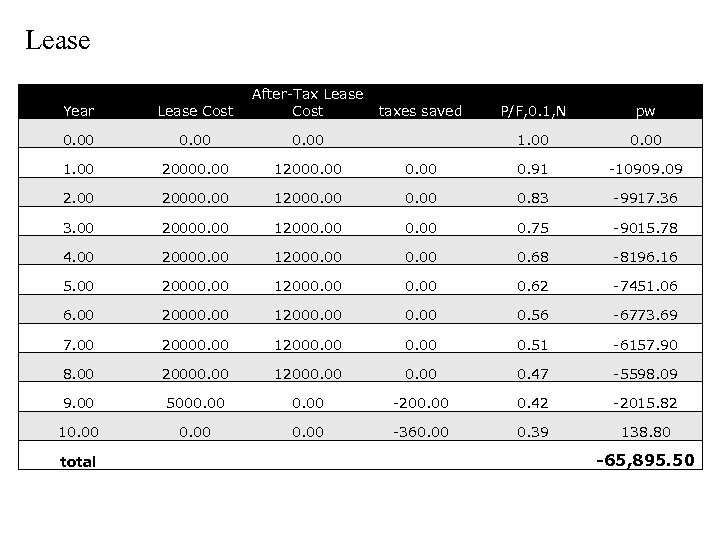Lease Year Lease Cost After-Tax Lease Cost 0. 00 1. 00 20000. 00 12000. 00 20000. 00 3. 00 P/F, 0. 1, N pw 1. 00 0. 91 -10909. 09 12000. 00 0. 83 -9917. 36 20000. 00 12000. 00 0. 75 -9015. 78 4. 00 20000. 00 12000. 00 0. 68 -8196. 16 5. 00 20000. 00 12000. 00 0. 62 -7451. 06 6. 00 20000. 00 12000. 00 0. 56 -6773. 69 7. 00 20000. 00 12000. 00 0. 51 -6157. 90 8. 00 20000. 00 12000. 00 0. 47 -5598. 09 9. 00 5000. 00 -200. 00 0. 42 -2015. 82 10. 00 -360. 00 0. 39 138. 80 total taxes saved -65, 895. 50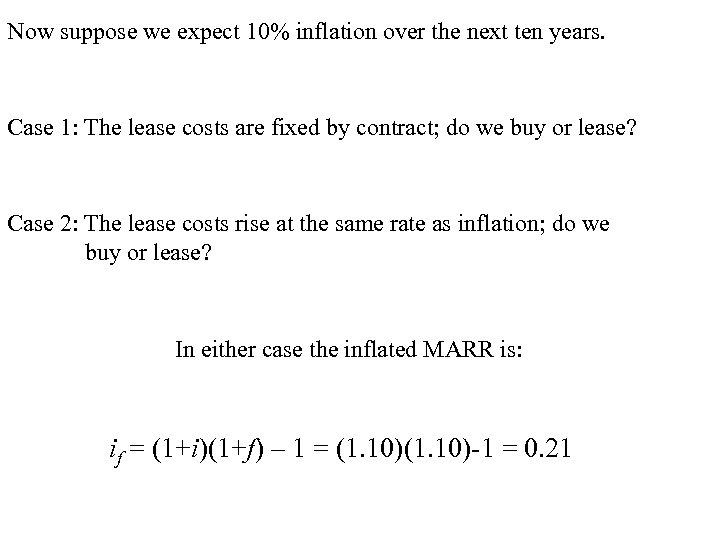Now suppose we expect 10% inflation over the next ten years. Case 1: The lease costs are fixed by contract; do we buy or lease? Case 2: The lease costs rise at the same rate as inflation; do we buy or lease? In either case the inflated MARR is: if = (1+i)(1+f) – 1 = (1. 10)-1 = 0. 21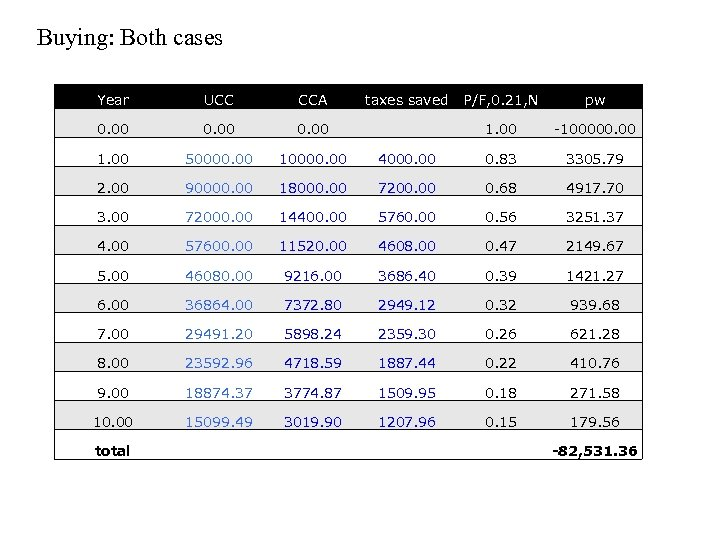Buying: Both cases Year UCC CCA 0. 00 1. 00 50000. 00 10000. 00 2. 00 90000. 00 3. 00 P/F, 0. 21, N pw 1. 00 -100000. 00 4000. 00 0. 83 3305. 79 18000. 00 7200. 00 0. 68 4917. 70 72000. 00 14400. 00 5760. 00 0. 56 3251. 37 4. 00 57600. 00 11520. 00 4608. 00 0. 47 2149. 67 5. 00 46080. 00 9216. 00 3686. 40 0. 39 1421. 27 6. 00 36864. 00 7372. 80 2949. 12 0. 32 939. 68 7. 00 29491. 20 5898. 24 2359. 30 0. 26 621. 28 8. 00 23592. 96 4718. 59 1887. 44 0. 22 410. 76 9. 00 18874. 37 3774. 87 1509. 95 0. 18 271. 58 10. 00 15099. 49 3019. 90 1207. 96 0. 15 179. 56 total taxes saved -82, 531. 36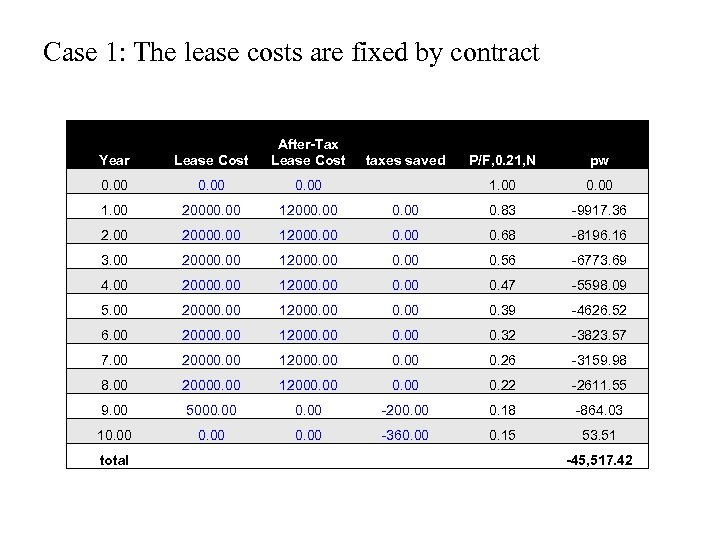Case 1: The lease costs are fixed by contract Year Lease Cost After-Tax Lease Cost 0. 00 1. 00 20000. 00 12000. 00 20000. 00 3. 00 P/F, 0. 21, N pw 1. 00 0. 83 -9917. 36 12000. 00 0. 68 -8196. 16 20000. 00 12000. 00 0. 56 -6773. 69 4. 00 20000. 00 12000. 00 0. 47 -5598. 09 5. 00 20000. 00 12000. 00 0. 39 -4626. 52 6. 00 20000. 00 12000. 00 0. 32 -3823. 57 7. 00 20000. 00 12000. 00 0. 26 -3159. 98 8. 00 20000. 00 12000. 00 0. 22 -2611. 55 9. 00 5000. 00 -200. 00 0. 18 -864. 03 10. 00 -360. 00 0. 15 53. 51 total taxes saved -45, 517. 42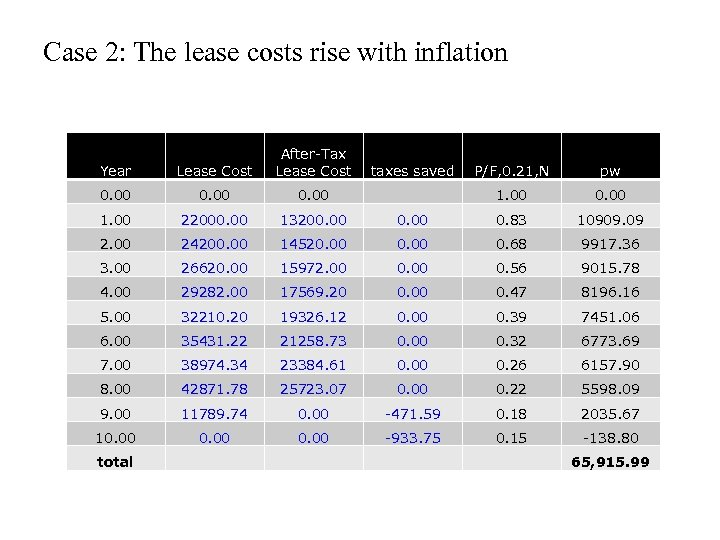Case 2: The lease costs rise with inflation Year Lease Cost After-Tax Lease Cost 0. 00 1. 00 22000. 00 13200. 00 24200. 00 3. 00 P/F, 0. 21, N pw 1. 00 0. 83 10909. 09 14520. 00 0. 68 9917. 36 26620. 00 15972. 00 0. 56 9015. 78 4. 00 29282. 00 17569. 20 0. 00 0. 47 8196. 16 5. 00 32210. 20 19326. 12 0. 00 0. 39 7451. 06 6. 00 35431. 22 21258. 73 0. 00 0. 32 6773. 69 7. 00 38974. 34 23384. 61 0. 00 0. 26 6157. 90 8. 00 42871. 78 25723. 07 0. 00 0. 22 5598. 09 9. 00 11789. 74 0. 00 -471. 59 0. 18 2035. 67 10. 00 -933. 75 0. 15 -138. 80 total taxes saved 65, 915. 99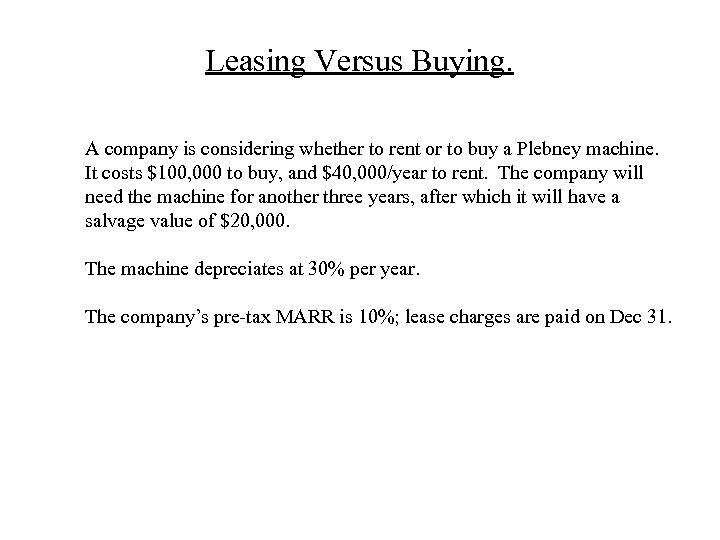Leasing Versus Buying. A company is considering whether to rent or to buy a Plebney machine. It costs \$100, 000 to buy, and \$40, 000/year to rent. The company will need the machine for another three years, after which it will have a salvage value of \$20, 000. The machine depreciates at 30% per year. The company’s pre-tax MARR is 10%; lease charges are paid on Dec 31.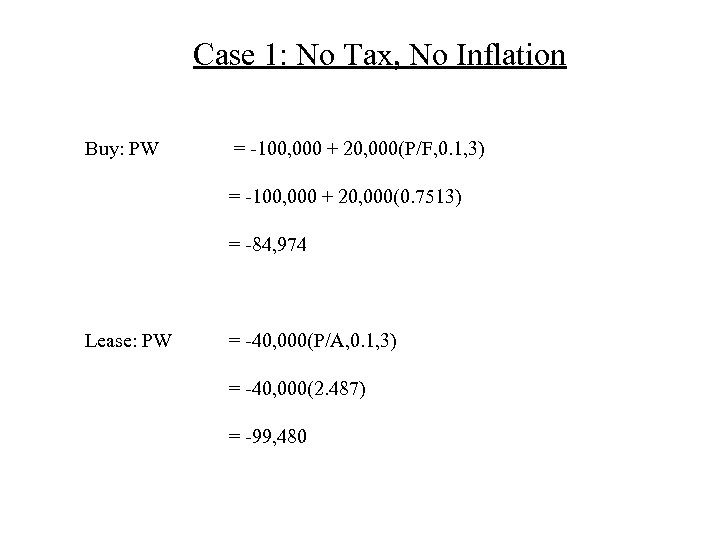Case 1: No Tax, No Inflation Buy: PW = -100, 000 + 20, 000(P/F, 0. 1, 3) = -100, 000 + 20, 000(0. 7513) = -84, 974 Lease: PW = -40, 000(P/A, 0. 1, 3) = -40, 000(2. 487) = -99, 480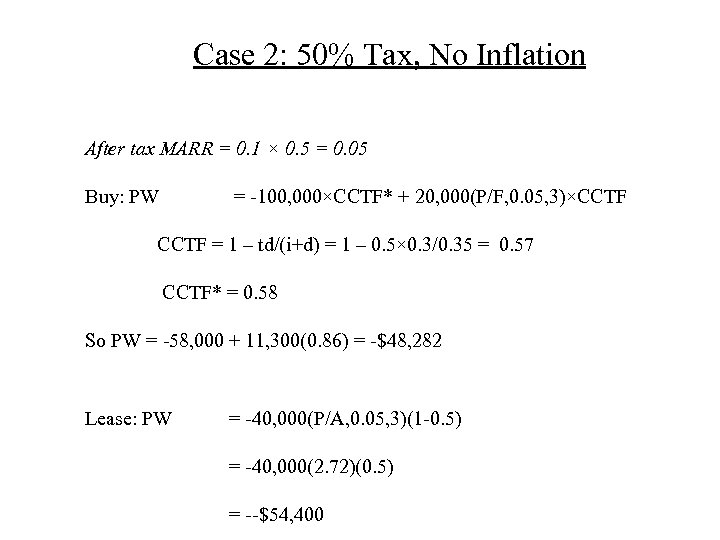Case 2: 50% Tax, No Inflation After tax MARR = 0. 1 × 0. 5 = 0. 05 Buy: PW = -100, 000×CCTF* + 20, 000(P/F, 0. 05, 3)×CCTF = 1 – td/(i+d) = 1 – 0. 5× 0. 3/0. 35 = 0. 57 CCTF* = 0. 58 So PW = -58, 000 + 11, 300(0. 86) = -\$48, 282 Lease: PW = -40, 000(P/A, 0. 05, 3)(1 -0. 5) = -40, 000(2. 72)(0. 5) = --\$54, 400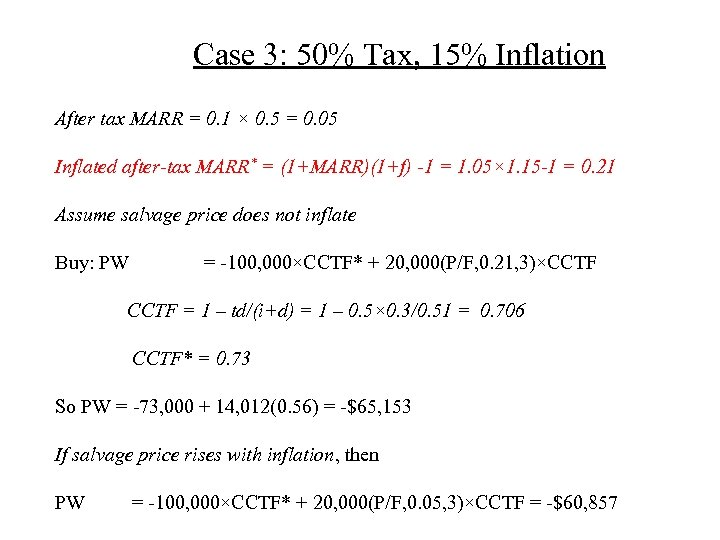Case 3: 50% Tax, 15% Inflation After tax MARR = 0. 1 × 0. 5 = 0. 05 Inflated after-tax MARR* = (1+MARR)(1+f) -1 = 1. 05× 1. 15 -1 = 0. 21 Assume salvage price does not inflate Buy: PW = -100, 000×CCTF* + 20, 000(P/F, 0. 21, 3)×CCTF = 1 – td/(i+d) = 1 – 0. 5× 0. 3/0. 51 = 0. 706 CCTF* = 0. 73 So PW = -73, 000 + 14, 012(0. 56) = -\$65, 153 If salvage price rises with inflation, then PW = -100, 000×CCTF* + 20, 000(P/F, 0. 05, 3)×CCTF = -\$60, 857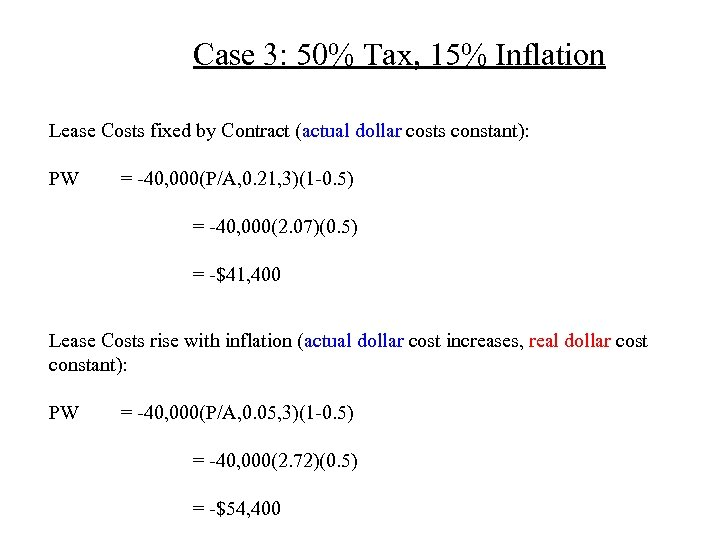Case 3: 50% Tax, 15% Inflation Lease Costs fixed by Contract (actual dollar costs constant): PW = -40, 000(P/A, 0. 21, 3)(1 -0. 5) = -40, 000(2. 07)(0. 5) = -\$41, 400 Lease Costs rise with inflation (actual dollar cost increases, real dollar cost constant): PW = -40, 000(P/A, 0. 05, 3)(1 -0. 5) = -40, 000(2. 72)(0. 5) = -\$54, 400